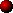ROTARY GAP ANALYSIS

This section discusses the factors influencing the design of a resonant charging system using a rotary spark gap. In particular it discusses how the following three variables interact:

• Rotary firing rate, (BPS)
• Tank capacitor value, (Cp)
• Ballast inductance value,

Various combinations of rotary speed, tank capacitor and ballast settings are explored and performance is characterised in terms of power throughput and power factor. Some thought is also given to peak capacitor voltage and the likelihood of saturation in the supply transformer.

This page contains theory about resonant charging and also includes simulation results for NST and power transformer based Tesla Coil systems. For those who are not interested in the theory, the graphs are at least worth some attention.

(Please note that all simulation results presented here refer to operation on a 50Hz supply.)

Introduction,

When a rotary spark gap is used in an AC system which employs resonant charging, there are three characteristic frequencies at work in the system:

• The AC supply frequency,

• This is the frequency of the mains AC supply voltage, (50Hz in UK and Europe, 60Hz in USA.)
• The rotary firing frequency, (BPS)

• This is the number of discharges per second that the rotary spark gap imposes on the system.
• The resonant charging frequency, (Fr)

• This is the natural resonant frequency of the charging circuit formed by the tank capacitor combined with the leakage inductance or ballast inductance of the supply.

The AC supply frequency is usually fixed. The actual value depending on which country you live in. However, the Tesla coil designer has considerable freedom in the choice of the rotary firing rate and the characteristics of the resonant charging circuit.

The rotary firing frequency can be an integer multiple of the supply frequency if a synchronous rotary is used, or can be totally unrelated to the supply frequency in the case of an asynchronous rotary. The asynchronous rotary represents the general case, whereas the synchronous rotary represents a special case. This page will cover a wide range of firing rates including some speeds that happen to be synchronous. Information presented here is therefore applicable to both Synchronous and Asynchronous systems.

The resonant charging properties depend on the chosen tank capacitor value and the leakage or ballast inductance of the supply. In the case of a system powered by a Neon Sign Transformer, the tank capacitor resonates with the leakage inductance built into the transformer. In the case of a system which is powered by an externally ballasted power transformer, the tank capacitor resonates with the ballast inductance which is "reflected" to the HV side of the step-up transformer.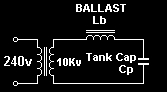Regardless of the type of power supply used, we can always reduce the circuit to simplified model shown opposite. This is done by imagining that the leakage inductance or ballast inductance is in series with the high voltage winding of the step-up transformer. We will call this Lb. Its value will typically be in the tens or hundreds of Henries.

This way of modelling the system is explained in more detail in previous sections on ballasting and resonant charging.

In summary this method allows us to reduce the charging circuit to a high voltage source connected to a series resonant circuit.

Dealing with the resonant charging circuit,

Although the resonant charging circuit consists of leakage inductance and tank capacitance, the actual L and C values are not the most useful parameters to discuss when considering the behaviour of the circuit. It is more useful to work with two other parameters of the resonant circuit which are derived from L and C:

• Natural resonant frequency,

• Fr = 1 / 2 pi sqrt (Lb Cp)

This is the resonant frequency of the charging circuit, and is inversely proportional to Lb x Cp. It defines all of the timing related behaviour of the resonant charging system such as the optimum rotary BPS, rotary phase, power factor, and resonant voltage rise. It specifically defines the shape of the charging waveforms.
• Characteristic impedance,

• Zr = sqrt (Lb / Cp)

This is proportional to Lb / Cp and determines the power throughput aspect of the charging circuit. Zr can be thought of as a kind of load impedance connected across the output of the HV transformer. A low Zr value implies high power throughput, and a high Zr implies low power throughput.

Rather than designing a charging circuit in terms of the tank capacitor Cp and ballast Lb, we should design it in terms of its resonant frequency Fr and characteristic impedance Zr, since these are the parameters which really define its behaviour. This implies manipulating Cp and Lb in conjunction, rather than adjusting one variable in isolation. Let me explain, by way of two examples…

If a particular system operates well with a given primary capacitor Cp and ballast Lb, we can easily double the power throughput by changing Cp and Lb in conjunction.

If Cp is doubled and Lb is halved, then the product Lb x Cp is unchanged and the resonant charging frequency Fr remains the same. This ensures that none of the timing aspects of the design change. Ie. The rotary speed and phase need not be changed, and the power factor and charging waveforms remain the same. However, the change in Lb / Cp means that the characteristic impedance of the circuit is halved. This implies a doubling in the power that is processed by the system, and an approximate 40% gain in spark length.

(The above analysis explains why doubling the tank capacitance is a wise decision when a second NST is added to a Tesla coil power supply. Paralleling two identical NSTs doubles the available current or effectively halves Lb. This means that Cp should be doubled in order to keep Fr the same. Only Zr is changed. The power throughput is doubled, but the spark gap still fires at the same rate, and voltages in the charging circuit remain unchanged.)

Similarly, if we want to operate a particular system at a higher rotary firing rate, but with similar power throughput, we can also do this by changing Cp and Lb in conjunction.

If Cp and Lb are both halved, then the resonant frequency is halved. This implies changes to the charging waveforms as the tank capacitor will now recharge much quicker following each firing. The optimum rotary speed will now be higher, and the power factor and peak voltages will also be different. However, since Lb / Cp remains unchanged, the characteristic impedance of the circuit is the same, and a similar amount of power will be processed at the new rotary speed. Such a change allows the merits of different rotary speeds to be compared without changing the overall power throughput greatly.

The graph below summarises the influence of Lb and Cp on the behaviour of the resonant charging circuit: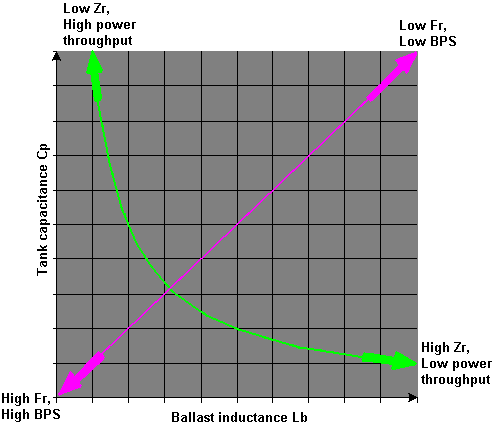It should be mentioned here that any change to the tank capacitance Cp implies that the tap point of the Tesla coil primary would need to be adjusted in order to regain correct tuning of the Tesla Coil as a whole.

Types of power supply,

In a system using an externally ballasted power transformer, the designer can readily change Lb by altering the inductance of the ballast inductor on the low voltage side of the transformer. The ballast inductance is transformed by the turns ratio squared and appears "reflected" at the high voltage side of the transformer.

Therefore, with an adjustable ballast and primary capacitor, both the resonant frequency of the charging circuit Fr and the impedance Zr can be altered independently as desired. This is a very flexible system indeed.

In systems based on shunted transformers such as Neon Sign Transformers the leakage inductance is most often fixed during the manufacture of the transformer. Due to this restriction, design methods involving shunted transformers and externally ballasted transformers are slightly different. The requirements of the design also become subtly different when moving from small shunted NST systems to higher powered externally ballasted transformers.Internally shunted supplies (NSTs)

Power supplies consisting of Neon sign transformers have internal magnetic shunts, which are built-in at the design stage. These shunts lower the coupling coefficient of the transformer and introduce a fixed amount of leakage inductance Lb in series with the secondary winding.

Since Lb is fixed, the designer can only influence the behaviour of the resonant charging circuit by changing the tank capacitor Cp. However, this has the undesirable affect that it changes both the resonant frequency Fr and the characteristic impedance Zr simultaneously ! This lack of flexibility has to be accepted with internally shunted transformers.

(Technically the designer could increase Lb by adding external ballast, or decrease Lb by paralleling several NSTs, although for this analysis we will assume that Lb is fixed.)

With Lb fixed, the variables in the system are the tank capacitor size Cp and the rotary firing rate BPS.
The objective of the analysis is to find the optimum capacitor size to obtain maximum power throughput at a particular rotary firing rate. Maximum power throughput results in the best spark performance.

The graph below was produced using the results from a large number of Microsim simulations. It shows the power throughput with various capacitor sizes at a variety of rotary firing rates . The simulation uses a 10kV/200mA NST model for the supply. (This has a leakage inductance of 159 Henries at 50Hz. The "Matched" capacitor size for resonance at the 50Hz line frequency would be 64nF.)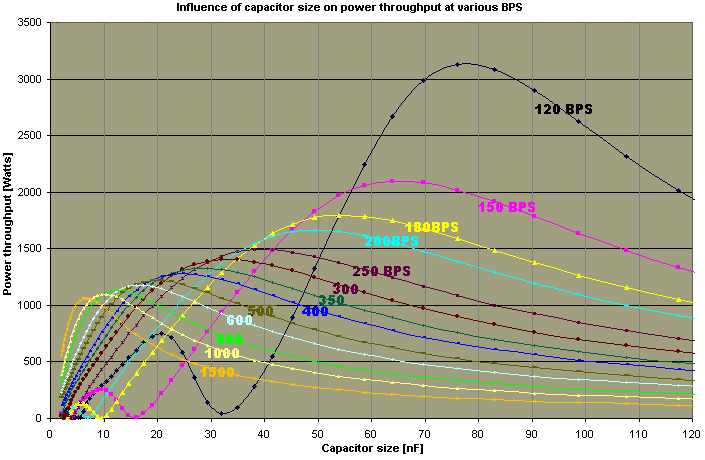Each coloured line represents operation at a particular rotary firing rate (BPS).

From the graph it can be seen that different rotary break rates give maximum power throughput with different sized tank capacitors. For example, operation at 150BPS (Magenta) requires a 65nF tank capacitor for maximum power, whereas operation at 1000BPS (Yellow) requires 10nF of tank capacitance for maximum power with this particular supply.

In general, low speed rotaries need large tank capacitors, and high speed rotaries need smaller tank capacitors to obtain maximum power throughput. However, it should be realised that higher speed rotaries give rise to progressively less power throughput even when the optimum tank capacitance is employed. Notice how the 150BPS line peaks at over 2000watts, whereas the 1000BPS line peaks at only 1100watts. This reduction in power throughput at high break rates is one clear disadvantage of using internally shunted transformers that have a fixed leakage inductance.

It is also evident that the 10kv/200mA NST supply is capable of delivering more than its rated 2kW power with low rotary firing rates. Unfortunately, the above graph is somewhat misleading in this respect as asynchronous operation between 100BPS and 200BPS is heavily plagued with surging problems. These problems arise due to beating between the firing frequency and the supply frequency. However, 100BPS synchronous operation is a highly workable firing rate. It allows more than the rated amount of power to be drawn from shunted transformers and displays no surging due to the synchronous operation.

Choice of primary tank capacitor,

Is there an easy way to calculate the best tank capacitor size for an NST system using a rotary gap ?

Tank capacitors in NST based systems are often compared to the "Matched" size required for resonance at the supply line frequency. For this particular 10kv/200mA supply the "matched" capacitor size is 64nF. This being the value which will resonate at 50Hz with the 159 Henry leakage inductance of the transformer. The graph below summarises the optimum capacitor sizes for a wide range of rotary firing rates. It also includes an approximate rule of thumb calculation to enable the best capacitor size to be found for a given firing rate.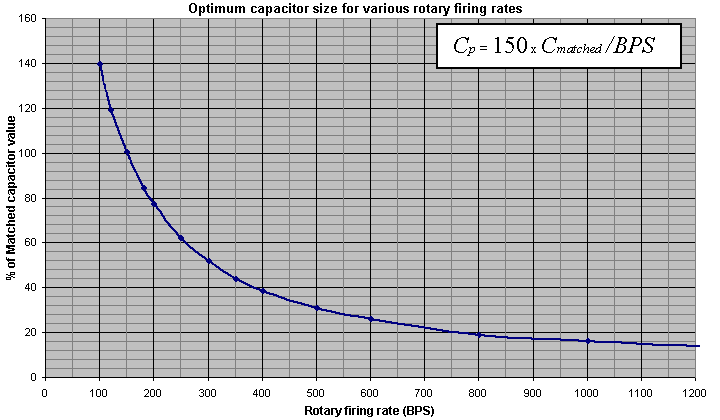The optimum tank capacitor Cp in an NST based system can be found from the following approximation:

C

p =
150 x Cmatched /BPS

Where: Cmatched is the "matched" capacitor value for resonance at 50Hz with the NST leakage inductance.

C

matched
= I / (V x 2 x pi x 50)

Example: Consider a 12kV/60mA neon sign transformer operating on a 50Hz supply.

The "matched" capacitor value is:

C

matched
= 0.060 / (12000 x 2 x pi x 50)

C

matched = 15.9nF

If a 100BPS synchronous rotary is to be used, then the required capacitance is found by making BPS=100:

C

p =
150 x 15.9 / 100

C

p = 23.9nF

or alternatively, if a 200BPS synchronous rotary is to be used, then the required Cp can be found by making BPS=200:

C

p =
150 x 15.9 / 200

C

p = 11.9nF

Other considerations,

The above analysis is concerned only with optimising the tank capacitor for maximum power throughput. This is usually the main objective since real power throughput is what produces long sparks. However, some thought should also be given to other parameters such as:

• VA draw,

• This is the product of the supply voltage and supply current, and gives an indication of the amount of current drawn from the supply cord. In an ideal system the VA draw would equal the real power throughput, (Power factor=1) In practice the VA draw is always higher than the real power throughput due to some reactive current flow.
• Power factor,

• This is equal to the power throughput divided by the VA draw of the Tesla coil. It can be thought of as a kind of measure of efficiency, as it indicates what proportion of the supply current actually goes into making sparks. An ideal system would have a power factor of 1, meaning that all of the supply current is processed as real power. Power factors of less than 1 imply a higher supply current in order to achieve the same power throughput. Therefore we usually seek to maximise the power factor in order to minimise heating in the transformer windings.
• Peak voltage,

• This is the maximum voltage to which the tank capacitor is charged, before the rotary fires. This voltage also appears across the output of the HV transformer. It is useful to know the peak voltage when specifying the tank capacitor or when considering the degree of overvoltage that the transformer will be exposed to in operation.
• Average voltage,

• This is the average voltage across the capacitor and HV winding of the step-up transformer. Since transformers saturate due to an excessive volt-second product, it is useful to know the average voltage across the transformer winding. (Actually the average of the magnitude of the high voltage, since the waveform is AC.)

The table below shows real power and VA draw relative to the face-plate VA rating of the NST. Peak voltage is relative to the normal peak open circuit voltage of the NST, and average voltage is relative to the NST's normal average secondary voltage. (For example, a 10kV/100mA NST is rated at 1kVA, its peak voltage is 14kV and its average voltage is 9kV.)

Values are given at various break rates with the optimum capacitor sizes shown. This allows the merits of various break rates to be compared. (Results for synchronous speeds assume that the rotary phase is set correctly.)

 Rotary speed Tank cap Cp (% of matched) Real power (% of va rating) VA draw (% of rating) Power Factor Peak voltage relative to o/c Avg voltage relative to o/c 100 BPS sync 150 % 103 % 111 % 0.92 147% 116 % 200 BPS sync 75 % 83 % 99 % 0.84 132 % 107 % 300 BPS sync 50 % 70 % 87 % 0.80 150 % 84 % 400 BPS sync 38 % 64 % 83 % 0.77 150 % 81 % 500 BPS sync 30 % 61 % 81 % 0.75 150 % 76 % 800 BPS sync 19 % 56 % 80 % 0.70 150 % 71 %

The average voltage column in the table shows that there is some possibility of transformer saturation if a 100BPS or 200BPS rotary is used. However, most transformers are probably capable of withstanding a few percent of overvoltage before saturation becomes problematic.

The affect of reducing the capacitor size at high rotary speeds is to increase the natural resonant frequency of the charging circuit. This makes the capacitor re-charge quicker, and tends to make the capacitor charging waveform become more spikey : lowering its average value, but increasing the peak value. (This is evident by looking down the last two columns of the table above.) A high peak voltage is important because this determines the energy stored in the tank capacitor before discharging. A low average value is also desirable because this reduces the likelihood of transformer saturation, and reduces the average voltage seen by the capacitor dielectric. Therefore, the spikey charging waveform is actually a good thing.

Two other things are apparent from the table above. Firstly, all of the examples indicate peak voltages in excess of the normal open circuit voltage of the transformer. This means that when the system is run at full power, the transformer secondary will be overvolted considerably due to the effect of the resonant rise. If this overvoltage cannot be accepted, the tank capacitance can be increased to load down the NST, and reduce the peak voltage. However, this comes at the cost of decreasing power throughput, and poorer power factor !

Secondly, many of the power factor values in the table are below 0.8. The power factor can be improved by decreasing the tank capacitance. Unfortunately this also reduces the overall power throughput, and causes the peak voltage to increase !!!

As always there is a trade-off, and this arises because the leakage inductance Lb in an NST based system is fixed.

In this case, a poor power factor is best dealt with by connecting some power factor correction capacitors across the supply line. In practice NST based systems are usually of relatively low power, so little PFC capacitance is required to cancel the small reactive current flow, and give a considerable improvement in the power factor.